# Artificial Intelligence - Part 2.1

## State-Space Search Methods

Posted by Utkarsh Jain on May 17, 2020 · 15 mins read

This is Part 2.1 in the Introduction to A.I. blog series. Read Part 1 for basic introduction to A.I.

## State-Space Search Methods

In the previous blog, I tried to introduce the concept of artificial intelligence, why it is needed and the different ways to achieve it. The main topics for this blog are:

Some of the most fundamental decision-making problems in A.I. can be reduced to the problem of searching for a path in a graph. The nodes of the graph represent the state (of the world, or some system), the arcs of the graph represent (instances of) different actions, and paths in the graph represent sequences of actions. Typically we are interested in paths that start from a given initial state and end in a goal state.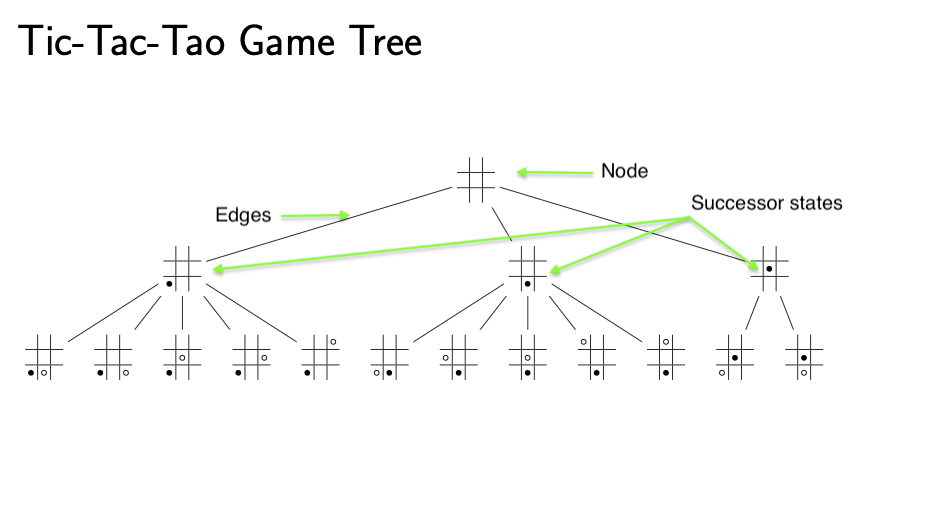When these graphs are small, the most basic graph algorithms, such as Dijkstra’s algorithm, can be applied. However, in all but the simplest A.I. applications, these graphs have a very large size, making the simplest and most direct ways of finding paths inapplicable. For example, even in very limited systems, like a deck of cards, the number of ways of combining the components of the system can be astronomic: a deck of 52 cards can be shuffled to 52! different orderings. A far higher number of configurations are possible in typical real-world systems. This combinatorial complexity is one of the main reasons for the difficulty of A.I. and the need to develop and deploy more complex programming technologies.

### Exploring Search State

Solving a search problem amounts to traversing some (abstract) search space to find a solution, or to spot that no solution exists. Of course, it depends on the problem of what a solution is, but it is typical that the search starts from a certain point in the space and then attempts to approach the point being searched for. In many cases, we are also interested in the route itself and it might form an essential part of the solution such as a plan of what actions to take in the future. Many search problems emerging in A.I. systems can be formalized as state-transition models where

• The system has (or is in) a known particular state.
• The system is predictable: for any state and any action possible in that state, there is only one possible successor state (determinism of actions), and there are no other causes of change in the system.
• The objective is to reach a state that belongs to a designated set of goal states.

The choice of actions is up to the agent(s) operating in the system. Different actions and events may lead the system to several successor states.

The two classes of algorithms that are used to traverse the search space are uniformed and informed search algorithms.

These algorithms can be understood as uninformed or blind search algorithms because they are just able to detect when a goal has been reached, but they have no idea in which direction the goal(s) are located, nor in how many steps they could be reached.

Below, I have provided a sketch of a generic search algorithm that visits nodes in the order determined by a chosen strategy and, if a node satisfying the goal condition is found, reports on how the node can be reached. Two key concepts underlying the algorithm:

1. While traversing the graph, the nodes are visited one at a time and we keep track of visited nodes to avoid loops in the search.
2. The expansion of a node means that each successor node is checked: if it has not been visited, it is visited now and recorded for further (recursive) expansion.

The preceding ideas reveal that we are preparing ourselves to deal with search spaces where a node can be reached through different paths. Let us then formulate the general search algorithm in more detail.

Pseudo Code:

Inputs: a directed graph with an initial state S, a goal state G, and an expansion strategy E Output: a solution path or failure

Mark S as visited and expand it.

While there are nodes n recorded for expansion:

1. Choose a node n for expansion according to E.
2. If n is a goal node, return the path from S to n as the solution path.
3. Expand n.

Return failure.

Search algorithms can be compared in terms of their properties.

• Completeness: An algorithm is complete if it is guaranteed to find a solution to a problem instance when there is one.
• Termination: An algorithm is terminating if, for all inputs, it will eventually either return a solution or tell that there are none.
• Optimality: An algorithm is optimal if it is guaranteed that a solution found by the algorithm is best possible (such as having the lowest possible cost).
• Time complexity: The amount of CPU time required.
• Space complexity: The amount of memory required.

Next, I will present two basic strategies for the exploration of search spaces. The heart of the strategy goes back to how nodes recorded for expansion are stored and, in case several nodes are waiting for expansion, which node is chosen for expansion. As regards storage, the relevant data structure is a queue and the type of the queue will fix the strategy as well as the resulting search algorithm:

1. A last-in-first-out queue (LIFO) also known as a stack gives rise to depth-first search (DFS).
2. A first-in-first-out queue (FIFO) yields breadth-first search (BFS).

I will illustrate these algorithms by a simple problem in which a robot is moving in a room.

Disclaimer: If you have no prior knowledge in graphs or trees, things will get trickier for you now onwards.

### 2.1 Depth-First Search (DFS)

DFS is an algorithm for traversing or searching a graph or a tree data structure. The algorithm starts at the root node (selecting some arbitrary node as the root node in the case of a graph) and explores as deep as possible in each branch before backtracking. In other words, once we start down a path, we don’t stop until we get to the end or the leaf node. Since DFS is based on a stack of nodes recorded for expansion, it will always expand the node found deepest in the search space first.

Below, the robot considers the possible actions in the order N, S, E, and W. This is also the order in which the nodes are pushed on the stack and, naturally, this will affect the order in which nodes are taken into consideration when the recursive expansions of nodes take place.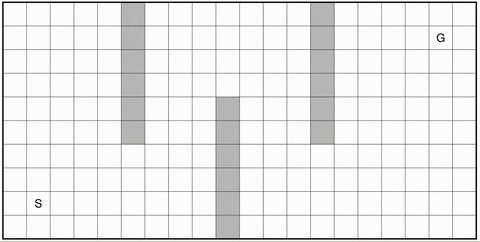In this case, the net effect of the ordering (N, S, E, W) is actually the reverse: the robot prefers to go west and, if that is not possible, east and, if that is not possible, south and finally, if that is not possible, north. Such a behavior can be recognized from the illustration above.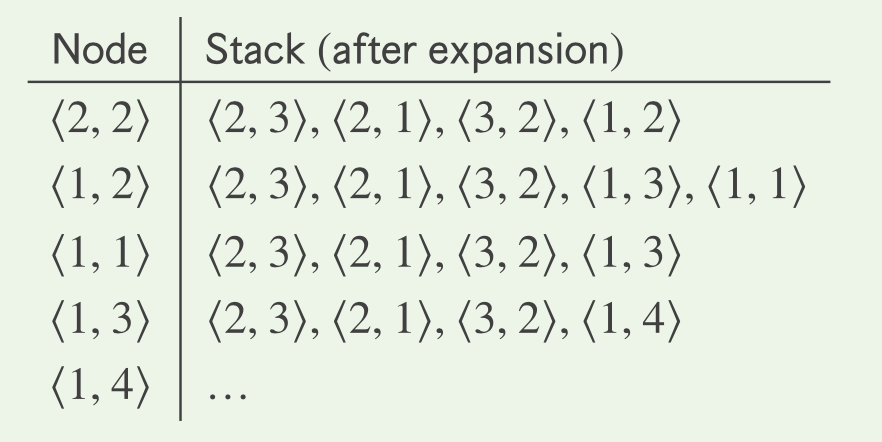Let us analyze the operation of the DFS algorithm and stacks resulting from expansions in more detail. The first step corresponds to the initialization of the algorithm from the source node S at coordinates <2,2> and it will visit the nodes <2,3>(for N), <2,1>(S), <3,2>(E), and <1,2>(W). These nodes will not be visited again. After the first expansion step, the node <1,2> appears on the top. It is popped from the stack and expanded next. As a result, two new nodes <1,3> and <1,1> and are visited next and pushed onto the stack. Thus <1,1> is on the top, but its expansion does not lead to any new nodes. The net effect is that it is simply removed from the stack, which reveals the node <1,3> for the next expansion. The only new node encountered is <1,4> (for N) and pushed onto the stack. Thus, it is the next target for expansion. In this way, the search proceeds until the goal node G at <19,9> is found. The solution path can be found by backtracking which is colored red in the final step of the animation.

The DFS algorithm is complete if loops are avoided. Otherwise, DFS might enter an infinite loop. In our formulation of the algorithm, we keep track of all visited nodes and hence avoid loops altogether. This might become unfeasible for large search spaces. It is easy to see from the figure that the path found by DFS is not the shortest possible and hence DFS is not optimal for our problem. The time and space complexities are bound by the size of the grid, i.e., they are linear in the size of the input graph (10*20 = 200 nodes). However, if the search space has not been completely enumerated, then time and space complexities are O(bm) and O(b*m) where b is the maximum branching factor and m is the maximum depth for encountering any node in the search space.

The preceding examples nicely illustrate that DFS may end up investigating irrelevant regions of the search space and, perhaps more seriously, may produce a suboptimal path to the goal node. By changing the type of the queue used to store nodes waiting for expansion, such behavior can be avoided and the quality of paths is improved accordingly. The FIFO used by BFS changes the behavior of our basic search algorithm so that nodes closest to the start node in the search space get expanded first.

For the sake of comparison, let us consider the actions of the robot in the order N, S, E, and W. The figure below illustrates how the search space is traversed by the BFS algorithm as well as the path found from S to G in red color.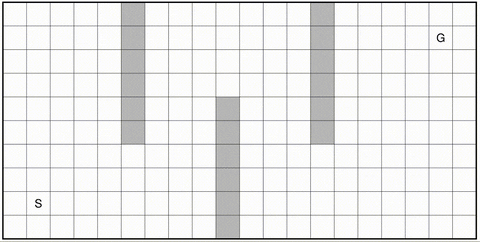This time, the net effect is that the robot would like to proceed to north and, if that is impossible, to the south and, if that is impossible, to the east and finally, if that is impossible, to west. The subsequent explanation discusses the progression of the search in more detail.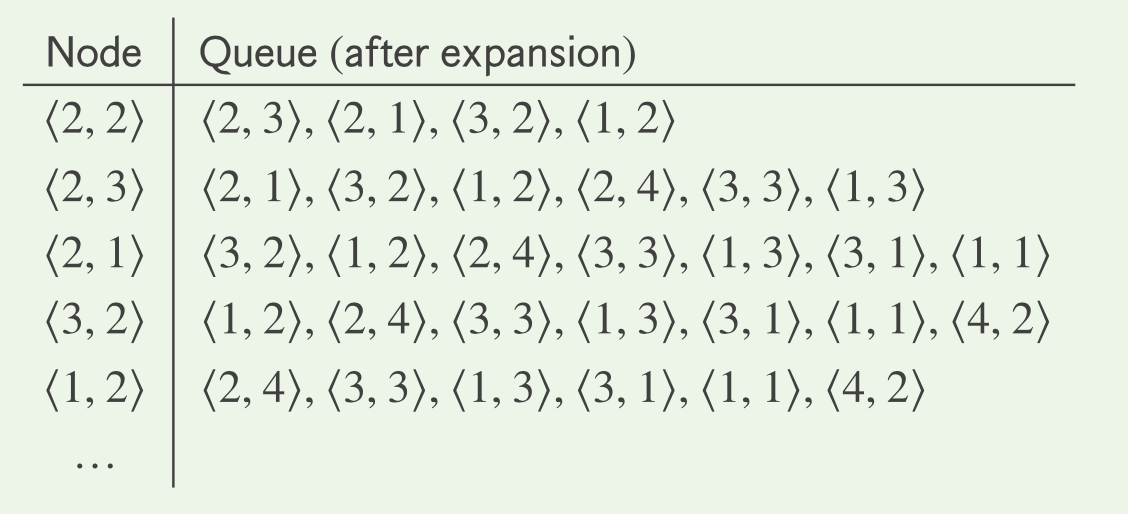The initialization of the search is identical to DFS, but after that, the search proceeds quite differently. Actually, the queue now contains the nodes within (Manhattan) distance 1 from the start node S and that set of nodes can be understood as the search frontier of BFS that will be expanded by nodes further and further away from S. Thus, the node <2,3> will be expanded next and this is how nodes <2,4>, <3,3>, and <1,3> are added to (the end of) the queue. When the next node, i.e., <2,1> , is expanded, two further nodes enter the queue:<3,1> and <1,1>. Similarly, the expansion of <3,2> enters <4,2> to the queue. No new neighbors are encountered when <1,2> is expanded. At this stage, the queue contains all nodes within (Manhattan) distance 2 of from S. In this way, the search proceeds until nodes with distance 30 get handled and the goal node G is found.

Like DFS, the BFS algorithm is also complete. It is also optimal. As regards the time complexity of BFS, it is analogous to that of BFS, i.e., it is O(bd) where b is the branching factor and d gives the depth of the (shallowest) solution in the search space. The space complexity is also O(bd) which is caused by the frontiers maintained by BFS. Their size may become exponential in the worst case because bd is exactly the number of leaves in a complete search tree of depth d and branching factor b.

### Summing Up:

We saw how we can use the basic uninformed state search algorithms to find a path from an initial state to a goal state. In the next blog, I will talk about how we can make these basic search algorithms a little more intelligent by giving them some form of “vision” with heuristic functions. I will talk about the Greedy Best-First Search and A* algorithm which is one of the most successful search algorithms to find the shortest path between nodes in a graph.

See you in the next blog!

Head to Part 2.2 for Informed Search Methods and Part 1 for basic introduction to A.I.

References:

• S. Russel and P. Norvig: Artificial Intelligence: A Modern Approach, Chapters 3 and 4, 3rd Edition, 2010.

Note: If you think there’s anything wrong in this article or something that can be improved in any way, feel free to point out in the comments.